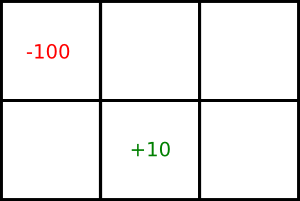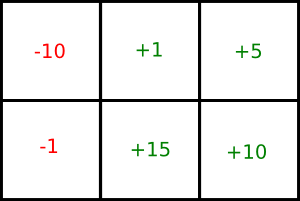Practice Exercise 9.C
Value Iteration

## 2: Learning Goals

• Use the value iteration algorithm to generate a policy for a MDP problem.
• Modify the discount factor parameter to understand its effect on the value iteration algorithm.
• Use the asynchronous value iteration algorithm to generate a policy for a MDP problem.

## 3: Directed Questions

1. What is the difference between infinite horizon and indefinite horizon problems? [solution]

2. What is the difference between fully observable MDPs and POMDPs? [solution]

3. What effect does decreasing the discount factor have on discounted reward? [solution]

4. Does a indefinite horizon stationary MDP have a stationary optimal policy? [solution]

5. In a stationary MDP, if an agent has a 20% chance of dying at each timestep, what is the optimal value for the discount factor? [solution]

## 4: Exercise: Gridworld

Consider the following grid world game:This is a stationary MDP with an infinite horizon. The agent can only be in one of the six locations. It gets the reward/punishment in a particular cell when it leaves the cell. It gets a reward of 10 for leaving the bottom-middle square and a punishment of 100 for leaving the top-left square. In each iteration of the game, the agent has to choose a direction to move. The agent can choose to move either up, down, left, or right. There is a 0.8 probability that it will move in that direction and a 0.1 probability that it will move in either of the neighboring directions. For example, if the agent wants to move up, there is a 0.8 probability that it will move up, a 0.1 probability that it will move left, and a 0.1 probability that it will move right. If the agent bumps into a wall, it stays in its current location and does not get any reward or punishment.
1. Perform one step of value iteration and show the resulting value function for each state. Use an initial value of zero in each state and a discount factor of 0.9. [solution]

2. What is the value in the top-left state after performing another step of value iteration? [solution]

3. What is the optimal policy? [solution]

4. What would the optimal policy be if the punishment in the top-left square was changed to each of the following values: 5, 0.0001, 0? [solution]

5. Suppose we have the following values for the states:If we select the top-left state and the action 'right', what is the value of Q[top-left,right] using asynchronous value iteration? Use a discount factor of 0.9 and the reward/punishment described in the original problem. [solution]

## 5: Learning Goals Revisited

• Use the value iteration algorithm to generate a policy for a MDP problem.
• Modify the discount factor parameter to understand its effect on the value iteration algorithm.
• Use the asynchronous value iteration algorithm to generate a policy for a MDP problem.